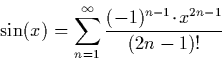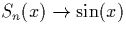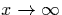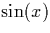Next: Cosine Up: Trigonometric Functions Previous: Trigonometric Functions

### Sine

The sine function is relatively simple to implement using the following approximation:Using this sum, we can easily construct a sequence S(x) such thatas. We by also computing a second sequence s(x), the current value of which can be computed as we generate the sequence when implementing this:

Observe that if x = 0, all terms of the sequence are zero. If x > 0, all even terms are greater than, and all odd terms less than it, and if x < 0, all even terms are less than, and all odd terms greater than it.

Using this information, it is extremely easy to generate a stream of nested intervals using two consecutive terms of the sequence of lower and upper bounds. Notice that the division is always by in integer. The integer division algorithm is simpler and quicker than algorithm for division of a real number by another real.

Martin Escardo
5/11/2000